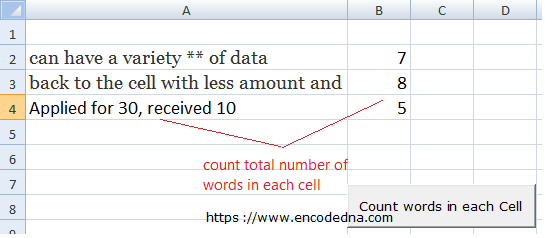﻿ Count Total Number of Words in a Cell or Range in Excel using VBA

# Count Total Number of Words in a Cell or Range in Excel using VBA

If you are using Excel extensively, then I am sure you have come across this situation or a similar situation at least once. Here in this article, I am going to share a simple method or code in Excel VBA on how to count total number of words in a cell or range in Excel.## Count Total number Words in a Cell without VBA

Excel does not provide an in-built function to count words in cells. However, there are methods to achieve this. If you are not using VBA, then you can use the below formula to find the total number of words in a cell.

=LEN(A2)-LEN(SUBSTITUTE(A2, " ","")) + 1

The above formula is written against a string of a values in the cell A2. For example, if A2 has a string of values, lets’ say, can have a variety of data, the formula will return a result of 6.

Talking about in-built functions, I have recently shared an article on a similar issue, where I have shown with an example on how to check if a cell or range has numbers using an in-built function called isNumeric() in VBA.

Now, lets see how you can do this in VBA.

## Count Total number Words in a Cell using VBA

Add a button (an ActiveX control) on your worksheet and write the below code.

```Option Explicit
Private Sub CommandButton1_Click()
countWords (Worksheets("Sheet1").Range("A2:A10"))
End Sub

Sub countWords(objRange As Range)

Dim cell As Variant
Dim element As Variant
Dim iCount As Integer
Dim iCnt As Long
Dim iRow As Long

iRow = 2
iCount = 1

For Each cell In objRange
For iCnt = 1 To Len(cell.Value)
element = Mid(cell, 1, InStr(cell, " "))

If Trim(element) <> "" Then
iCount = iCount + 1
objRange.Cells(iRow - 1, 2) = iCount
End If

cell = Replace(cell, element, "")
Next iCnt

iRow = iRow + 1
iCount = 1          // re-assign the counter.
Next cell
End Sub```
The button’s click event calls a procedure named countWords(). It takes a parameter in the form of a range. You can extend your range if you want.

Also Read: Count Total Number of Characters in a Cell in Excel

The procedure above runs two loops. The first loop gets a string value from each row. The second loop reads each element (words and other characters) in the string.

The Mid() function extracts a word from the string and add 1 to the counter (iCount). Finally, I am using the Replace() function to replace the extracted word from the string. The loop runs until it reaches the last word in the string.